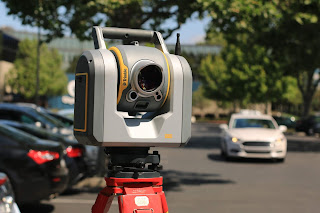### Surveying - Definition ,Principle and Classification

Surveying can be defined as the art of determining the relative positions of a point on or below or above the earth's surface by undergoing direct or indirect measurements of distance, elevation, and direction.Surveying - Definition, Principle, and Classes

## Significance of Surveying

Both Angular and Linear Measurements are determined in order to establish the points. The main objective of surveying is to prepare a map or plan that represents an area held on a horizontal plane.

• The horizontal projection of an area is represented in the plan and maps
• Vertical distances between the points are shown through contour lines, hachures, and other methods in vertical sections.

## Principles of Surveying

The two main principles of surveying are:

1. Working from whole to part
2. Locating a point by measuring from two points of reference
No Time to Read??? Watch Video on Principles of Surveying Here👇👇👇👇👇

### 1. Working From Whole to Part

This is the ruling principle that is used for both plane surveying and geodetic surveying. The whole to part principle of surveying is a method that initially starts with setting or establishing control points by less precise methods and further details are established by minor control points.

For example, If we need to survey a line AB as shown in figure-1 below, we can divide this line into parts as AC,CD,DE,EF,FB. We can start the measurement with AC, then CD, DE, EF and finally FB. This is called part to whole method. The issue with this method is that, as we move forward, from AC, if there arises any error, that gets accumulated and would be reflected in CD DE, EF and AB measurement. If AC is measured right, and there comes any error in CD or any following parts, the succeeding measurements will go wrong. So, instead of AB, our measurement will be AB' which is incorrect.

So, we opt the whole to part method. In which, we start from A and B to inwards. We initially measure AC, then BF, then CD, then FE and DE. If there comes any possibility of error in any of the measurement, it is not accumulated and  helps to localize the error as shown in figure-2. These are the main advantages of whole to part method.

### 2. Locating a Point by Measuring From Two Points of Reference

This principles states that any point must be measured with two points of reference which are the points that are already determined by standard measurements and they are fixed.

This can be explained with the following examples, where the points  P and Q are already fixed by knowing the distance PQ. If so, a point R can be obtained by the following options:
1. The distance PR and QR are measured. The point R is located by swinging the measured arcs to the same scale to which PQ was plotted. This is commonly used in chain surveying.2. The second option is by dropping a perpendicular from R to the reference line PQ, after which the length PS and SR are measured. The point R can be plotted using a set squares. This is commonly used for defining the details on the way of surveying measurements.
3. Third option is to obtain distance QR and angle PQR. This is used in traversing.
4. In the next method, the angles RPQ and RQP are measured, using an angle measuring instrument. It is widely used in triangulation.
5. Another option is to plot R by plotting RQP and PR. This is used in traversing and used less.

## Classes of Surveying

Primarily surveying can be classified into two classes:
1. Plane Surveying
2. Geodetic Surveying

#### Plane Surveying

In this type of surveying, the spheroidal shape of the earth is neglected. It considers the mean surface of the earth as a plane. Triangles that are formed by the survey lines are considered plane triangles. Level lines are straight and plumb lines are parallel.

#### Geodetic Surveying

In this type of surveying, the shape of the earth is taken into consideration. Lines laying on the earth's surface are curved lines and the respective triangles formed are spherical triangles. Hence, this surveying takes into consideration, spherical trigonometry.